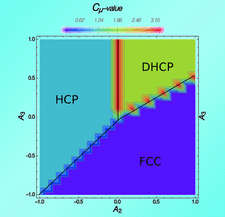issue contentsFOUNDATIONSADVANCES
ISSN: 2053-2733

September 2017 issueCover illustration: The field of computational mechanics allows order and disorder in crystallography to be treated on an equal footing. Ordering is viewed as an information-processing dynamic capable of storing, transmitting and processing information. Statistical complexity, a measure of the resources used by the computational system, is plotted as function of the interaction parameters in a finite-range model describing polytypism in close-packed structures. Interaction is taken to occur between actual layers of the stacking arrangement. [Rodriguez-Horta et al. (2017). Acta Cryst. A73, 377-386].

obituaries

Philip Coppens (1930–2017)

Obituary for Philip Coppens.

Davide Viterbo (1939–2017)

Obituary for Davide Viterbo

research papers

Close-packed structure dynamics with finite-range interaction: computational mechanics with individual layer interaction

This is the second contribution in a series of papers dealing with dynamical models in equilibrium theories of polytypism. Instead of using an Ising model over spins defined by the Hägg coding, a Hamiltonian considering direct interaction between the close-packed layers is assumed. The results of the phase diagram and the appearance of disorder are compared with the previous analysis using the Ising model. Computational mechanics is the framework under which the analysis is performed.

Mathematical aspects of molecular replacement. IV. Measure-theoretic decompositions of motion spaces

The minimal-volume search space in molecular replacement can be expressed in multiple ways wherein there is a trade-off between the sizes of rotation and translation subspaces. In particular, if the space group is a semi-direct product of a point group and a Bieberbach group, then the search space can be represented as a product of two manifolds: a spherical space form and a Euclidean space form.

The wavevector substar group in reciprocal space and its representation

A new concept of the wavevector substar group and its representation is established.

Real-time detection and resolution of atom bumping in crystallographic models

An O(n log n) algorithm that detects atom bonding in a unit cell is presented. As an application of this algorithm, an evaluation function for atom bumping is proposed, which can be used for real-time elimination of crystallographic models with unreasonable bond lengths during the procedure of crystal structure determination in direct space.

short communications

Accelerated scattering of convex polyhedra

The formulas for minn and maxn names in the classes of convex n-acra, as well as asymptotic relationships (as n → ∞) between them, are found. These explain the distribution of [minn, maxn] ranges on the real line.
Follow Acta Cryst. A